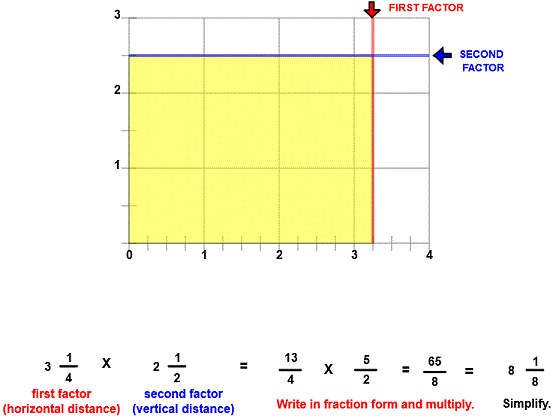# Multiply mixed numbers

## Multiply Mixed Numbers and Write in Lowest Terms

MULTIPLY MIXED NUMBERS
CORRECT:
ATTEMPTS:
SCORE:
PERCENT
WHOLE
NUMERATOR
DENOMINATOR

# MULTIPLY MIXED NUMBERS INSTRUCTIONS

Follow the directions in the dialog box after pressing the <START> button. The <EXPLAIN> button may be pressed after you enter the second factor to see how to do the example.

The following image was made fromMultiply Fractions-Strict is similar to the previous program MULTIPLY FRACTIONS except that both factors may be mixed numbers and the product must be written as a mixed number or whole number and in lowest terms.

See the program MIXED NUMBERS for information on writing fractions in mixed number form.

See the program RENAME IN LOWEST TERMS for information on writing fractions in lowest terms.

The above image shows six whole units.There are also three 12 units, two 14 units and one 18 unit. This all adds up to the product of 818 units.

You can see from the picture that the first factor is 314 units in length and the second factor is 2 12 units in length. To calculate the product, first write each factor in fraction form. Then multiply the numerators of each factor for the numerator of the product and the denominators of each factor for the denominator of the product.

When the program starts, you will be asked to identify the first factor and then the second factor. You will then be asked to find the product.

How to Cancel will play a short animation that demonstrates this shortcut way to write a fraction in lowest terms.

For more instruction on multiplying fractions go to How To Multiply Fractions.

After you enter the product you may press the <REPORT> button. The report will ask for your name but you may submit a code for your name. This report will give same results as on the dialog box. The report may be printed or e-mailed.